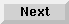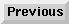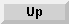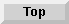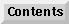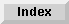7 Subprograms

## Multiple-Line Function Subprograms

The multiple-line function subprogram is used to define a numeric or string function and return a value to the calling program segment. The first line of a numeric multiple-line function subprogram is:

DEF FN subprogram name [(formal parameter list)]

For a string function, the first line is:

DEF FN subprogram name\$ [(formal parameter list)]

The subprogram name must be a valid name.

The last line in a multiple-line function subprogram should be:

FNEND

The value to be returned to the calling program segment as the value of the function is specified by:

RETURN numeric expression

or

RETURN string expression

The function subprogram is called automatically by specifying the function name and pass parameter list in a program line:

FN subprogram name [(pass parameter list)]

or

FN subprogram name\$ [(pass parameter list)]

Here is an example of a numeric function:

```10   DIM C(50)
20   A=10
30   B=20
40   FOR I=0 TO 50
50     C(I)=I
60   NEXT I
70   X=FNTotal(A,B,C(*))
80   PRINT "RESULT=";X
.
.
120  END
130  DEF FNTotal(X,Y,Z(*))
140     Tot=0
150     FOR I=X TO Y
160       Tot=Tot+Z(I)
170     NEXT I
190     FNEND

RUN
RESULT= 165
```
The function subprogram computes the value of Tot using the equation: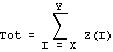Notice that the variable X in the function subprogram is not the same as X in the main program.

Here is an example of a string function:

```10   A\$="HELLO"
20   B\$="GOODBYE"
30   Rep=2
40   PRINT FNResult\$(A\$,B\$)
50   END
60   DEF FNResult\$(H\$,G\$)
70      String\$=H\$"..."G\$
80      RETURN "****"TRIM\$(String\$)"****"
90      FNEND

RUN
****HELLO...GOODBYE****
```
There can be more than one RETURN statement in a function subprogram, but only one is executed each time the subprogram is executed. Here is an example based on the previous numeric function subprogram:

```10   DIM C(2,2)
20   C(0,0)=C(0,1)=C(1,0)=C(1,1)=2
30   A=B=4
40   PRINT "RESULT=";FNTotals(A,B,C(*))
50   END
60   DEF FNTotals(X,Y,Z(*))
70     A=Z(0,0)+Z(0,1)+Z(1,0)+Z(1,1)
80     B=X+Y+A
90     IF XY THEN RETURN B
100    RETURN 2*B
110    FNEND

RUN
RESULT= 32
```
If a single-line and multiple-line function are defined with the same name and the name is referenced, the single-line function is the one that is accessed if it is defined in the calling program segment.

Eloquence Language Manual - 19 DEC 2002